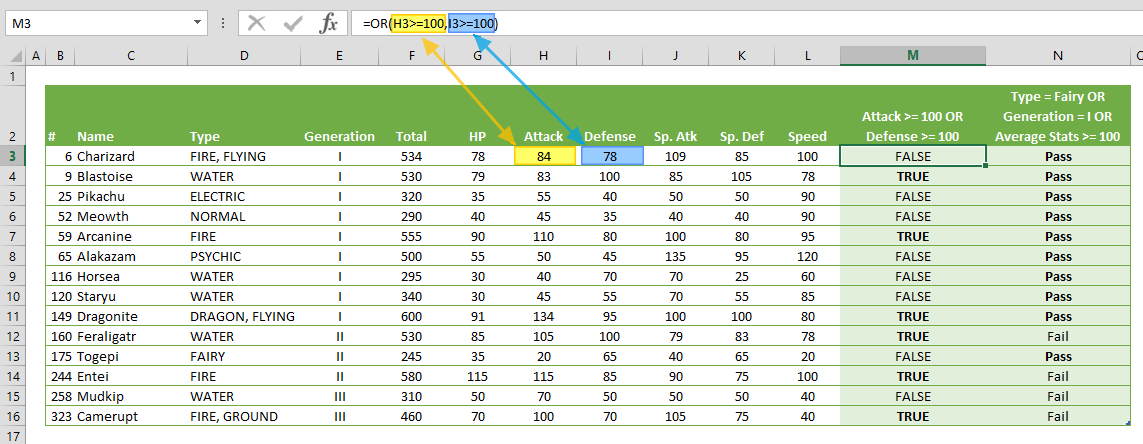The Excel OR function is a comparison function that returns TRUE if at least one of the specified conditions is TRUE. If no condition is met (FALSE), the return value is FALSE. In this guide, we’re going to show you how to use the Excel OR function and also go over some tips and error handling methods.

# Supported versions

• All Excel versions

# Syntax

OR(logical1, [logical2], …)

# Arguments

 logical1 The first condition or logical test. logical2 Optional. Additional conditions, up to maximum of 255 conditions.

Examples

## Example 1

=OR(84>=100,78>=100)
formula evaluates each test case, and returns FALSE since both tests return FALSE.## Example 2

=IF(OR("FIRE, FLYING"="Fairy","I"="I",AVERAGE({78,84,78,109,85,100})>=100),"Pass","Fail")
returns Pass because the second test ("I"="I") returns TRUE. Like the AVERAGE function, the OR function can be used in conjunction with other functions to produce different scenarios.# Tips

• Cheat sheet
 Calculation Returns TRUE OR TRUE TRUE TRUE OR FALSE TRUE FALSE OR FALSE FALSE
• Use the Excel OR function to extend the capabilities of other functions that use logic tests for parameters, like the IF function.
• Combine with other logical functions (AND, NOT, XOR) to generate more complex cases.
• Comparison operators:
 Operator Description Criteria Sample Criteria Meaning = Equal to “=10000” Equal to 10000 <> Not equal to “<>10000” Not equal to 10000 > Greater than “>10000” Greater than 10000 < Less than “>10000” Less than 10000 >= Greater than or equal to “>=10000” Greater than or equal to 10000 <= Less than or equal to “<=10000” Less than or equal to 10000

# Issues

## #VALUE!

Excel OR function returns a #VALUE! error if an argument doesn't provide a Boolean value.

## Empty cells

Empty cells are ignored by the function.